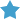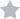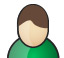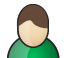# Dynamic Process v.2Simulation (5) |• Author
• Rating
• Type
• Revision
• Updated
• Richard Herz
•• Stack
• 7
• 1415 Times
• 15 September 2009
Example of a dynamic, interactive simulation of a physical process
This is an example of a dynamic, interactive simulation of a physical process. What is important are the scripting strategies to synchronize the objects which participate in the simulation. The actual process illustrated is not important. The process is water flow through a tank, with the water level in the tank controlled by a PI process controller (Proportional and Integral modes).

This is version 2. Version 2 improves on the strategy in the first version and adds synchronization between real time and simulation time.Richard HerzDec 4, 2015
This stack solves coupled, first-order, Ordinary Differential Equations (ODEs) by stepping in time (the independent variable here) with the Euler method. This type of system is termed an initial value problem.
The active process units are represented by button objects containing script. Here, these are tank, level indicator, valve, controller, and strip chart. The last three buttons listed are hidden from user view.
Each active process unit in the simulation contains one or more ODEs. For example, the tank unit (blue button that changes height) has this line in its updateState command:
add gDt*(1/tAx)*(tFlowIn - tFlowOut) to tLevel
The effect is to add a change in water level, ∆(Level), to the level: add (∆t * d(Level)/dt) to Level, where ∆t is a finite time step (the value of the global variable gDt) and where the ODE is d(Level)/dt = (1/tAx)*(tFlowIn - tFlowOut). The updateTrigger command in the stack script causes the updateProcess command in the stack script to send a periodic updateState command to all units.
The stack is designed such that active process units, and their ODEs, can be added or subtracted to or from the system model easily. Thus, the same structure and logic can be extended to simulate any initial value problem.
The logic in the updateTrigger command in the stack script is somewhat complex because it allows the time step size to be changed between any two updateProcess calls, while keeping correspondence between simulation time and real time. The logic can be simplified if one allows the time step size to be changed only after an updateDisplay. Changes in time step size save computation time when rates of change of system variable values change significantly during the solution.
The controller is a simplified version of the controller present in SimzLab's Control Lab simulations. SimzLab can be downloaded from the ReactorLab.net download page.
The Euler method introduces systematic errors into the solution. This is often not critical for illustrating concepts. In other simulations, we have made corrections for this such that the simulation reaches the exact solution after input parameter changes (Reactor Lab, Division 3 Thermal Effects, Lab 4 Dynamic CSTR). This is done by solving for the new steady-state solution of the nonlinear problem after an input change, then getting decay time constants from solution of the problem that has been linearized about the new nonlinear solution. Finally, the Euler solution is forced to the new steady state solution gradually at a rate given by the decay time constants.